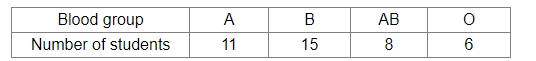# In a medical examination of students of a class, the following blood groups are recorded:

Question:

In a medical examination of students of a class, the following blood groups are recorded:From this class, a student is chosen at random. What is the probability that the chosen student has blood group AB?

(a) $\frac{13}{20}$

(b) $\frac{3}{8}$

(C) $\frac{1}{5}$

(d) $\frac{11}{40}$

Solution:

(c) $\frac{1}{5}$

Explanation:
Total number of students = 40
Number of students with blood group AB = 8

Let E be the event that the selected student's blood group is AB.

$\therefore$ Required probability $=P(E)=\frac{8}{40}=\frac{1}{5}$